# Lattice distribution

(diff) ← Older revision | Latest revision (diff) | Newer revision → (diff)

A discrete probability distribution concentrated on a set of points of the form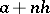, where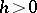,is a real number and. The numberis called the step of the lattice distribution, and if for no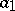and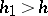the distribution is concentrated on a set of the form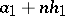,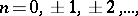thenis called the maximal step. An arithmetic distribution is a particular case () of a lattice distribution.

For a probability distribution with characteristic functionto be a lattice distribution it is necessary and sufficient that there exists a real number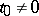such that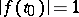; in this caseis the maximal step if and only if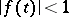forand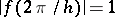. The characteristic function of a lattice distribution is periodic.

The inversion formula for a lattice distribution has the form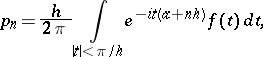where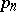is the probability that the lattice distribution ascribes to the pointand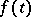is the corresponding characteristic function. The following equality also holds: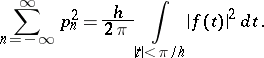The convolution of two lattice distributions with stepsand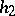and with finite supports is a lattice distribution if and only if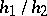is a rational number.

In the study of the limit behaviour of sums of independent random variables, the basic result of the central limit theorem on convergence towards the normal distribution is considerably complemented by local theorems for lattice distributions. The simplest example of a local theorem for lattice distributions is the Laplace theorem, which can be generalized as follows: Let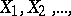be a sequence of independent identically-distributed random variables with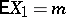,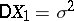, and let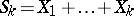while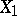takes values of the form,. Put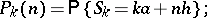for the asymptotic relation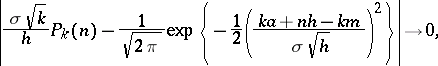as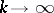, to be true uniformly with respect to, it is necessary and sufficient that the stepis the maximal step.

How to Cite This Entry:
Lattice distribution. Encyclopedia of Mathematics. URL: http://encyclopediaofmath.org/index.php?title=Lattice_distribution&oldid=11279
This article was adapted from an original article by N.G. Ushakov (originator), which appeared in Encyclopedia of Mathematics - ISBN 1402006098. See original article